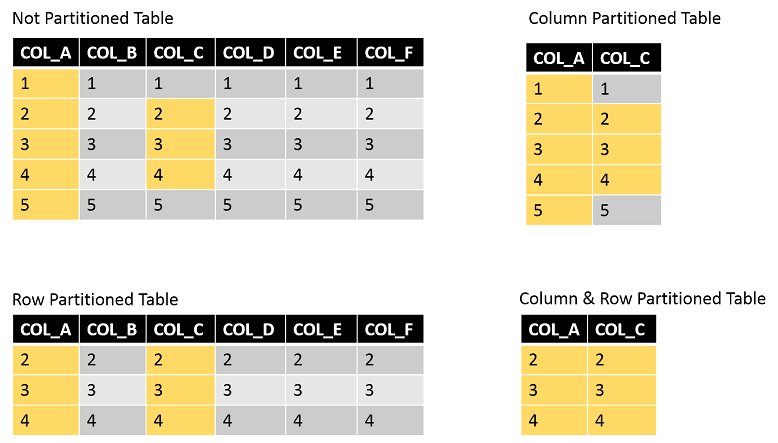# Teradata Partitioning – Row vs. Column

By Roland Wenzlofsky

December 21, 2014

## Teradata Partitioning – Row vs. Column

Column and row partitioning are two different methods of partitioning tables to minimize data access resource usage.

Row partitioning is the horizontal partitioning of tables, allowing the optimizer to limit data access to a cluster of table rows. Column partitioning is the vertical partitioning of tables, allowing the optimizer to restrict data access to a subset of table columns.

The rows of row partitioned tables are stored in physical partitions, which are clusters of rows. A cluster could, for example, holds all rows of a particular date.

Suppose such a cluster is used in the where condition of a query. In that case, the Teradata optimizer creates an execution plan, limiting access to the partitions containing only table rows with the date being searched for, and a full table scan (FTS) is avoided.

The storage of row partitioned tables is quite similar to the storage of not partitioned tables. The table’s rows are distributed across all AMPs and ordered by the partition number, the row hash value, and the uniqueness value. Not partitioned tables are internally stored in the same way as partitioned tables. All rows of a not partitioned table are considered to belong to the dummy partition 0.

When dealing with row partitioned tables, each table row is stored in one physical row on disk.

When using column partitioning, one or more table columns are building a cluster. Its partition represents each cluster. Column partitioned physical rows contain only a subset of columns and are not representing the complete logical rows.

While row partition clustering can be based on complex expressions, column partitioning is limited to the columns’ definition, building one cluster. Hence, from an implementation point of view, column partitioning is simpler.

The key benefit of row partitioned tables is partition elimination. For a query, selecting only an individual partition, the optimizer does not have to do a full table scan but can limit the search activity to the accessed partition.

The main benefit of column partitioned tables is selecting only a subset of columns, reducing disk IO.

The advantage of resource usage can be increased by combining column and row partitioning in one table.

Let me show you an example to analyze the four possible table setups (no partitioning, row partitioning, column partitioning, row and column partitioning together):

CREATE SET TABLE THE_TABLE (COL_A INTEGER, COL_B INTEGER, COL_C INTEGER, COL_D INTEGER, COL_E INTEGER, COL_F INTEGER);

We are executing the following query:

SELECT SUM(COL_A) FROM mytable WHERE COL_C IN (2,3,4);

In our example, row partitioning will be applied to column COL_C, and we assume column containers are available at least for the columns COL_A and COL_C:• In the case of a column-partitioned table, all five rows, but only two columns have to be accessed.
• In the not partitioned table, all five rows and all columns have to be accessed.
• In a row partitioned table, all columns, but only three rows have to be accessed.
• In a row and column-partitioned table, only three rows and only two columns have to be accessed.